abstractmath.org 2.0
help with abstract math

Produced by Charles Wells     Revised 2017-03-01

Introduction to this website    website TOC    website index

blog    Back to head of Functions chapter



### Contents

Math Object

Formula

Process

Dependency relation

Symmetries

Maps

Structure-preserving functions

# IMAGES AND METAPHORS FOR FUNCTIONS

#### A mathematician's mental representation of a function is generally quite rich and may involve many different metaphors and images kept in mind simultaneously.

By "images and metaphors" I am referring to the conceptual metaphors that mathematicians use in thinking about mathematical objects. Below are some references for images, metaphors and related ideas:

The illustrations were created using the Mathematica Notebooks Functions and Shapes.nb, FunctionDigraphForImMetFuncs.nb, and AnotherFamiliesFrozen.nb. These notebooks contain many more examples of the ways functions can be represented than are given in this article. For information about how to use them, read the abmath section Mathematica.

Like everything on abstractmath.org, the notebooks are covered by a Creative Commons ShareAlike 3.0 License.

## A function is a math object

#### Most basic mental representation: A function is a mathematical object.

This concept is discussed in Specification and Definition of Function as well as here.

• You must think of functions as math objects when you are taking the rigorous view, which happens specifically when you are trying to prove something about a function.
• Experienced mathematicians studying a function will think in terms of many different metaphors and images of the function, but will often return to the rigorous math object point of view, particularly when they are confused.

#### Like math objects, functions can have properties.

An integer can be odd, or a prime. A triangle can be isosceles, or obtuse. Like any math object, a function can have properties too.

• The function $x\mapsto x^2:\mathbb{R}\to\mathbb{R}$ (this definition uses barred arrow notation) is continuous and differentiable.
• The function $n\mapsto n^2:\mathbb{N}\to\mathbb{N}$ is injective but not surjective.
• The function defined by $f(x):=2{{\sin }^{2}}x-1$ is periodic with period $\pi$, meaning that for any $x$, $f(x+\pi )=f(x)$.   It is the function itself that has period $\pi$, not any value of it.

#### You can apply operations to many functions.

You can bisect an angle or add two numbers. In the same way you apply operations to functions.

Let $f(x):=2\sin^2x-1$ and $g(x):=x^2+2x-8$.

• You can multiply a real valued function by a number. For example, $2f$ is the function $x\mapsto 4\sin^2x-2$
• You can add two real valued functions. $f+g$ is the function $x\mapsto 2\sin^2x+x^2+2x-9$ There are more examples like this in the Wikipedia article pointwise.
• But you can’t do arithmetic operations to functions that don’t have numerical input and output. The database function$\text{Employee} \mapsto \text{Department}$that maps each employee of a company to the department they work in can't be added to the function $x\mapsto x^2$.
• You can differentiate and integrate $f$. But there are also plenty of functions you can’t differentiate or integrate, for example the Dirichlet function or any finite function.

#### A function can be an element of a set.

One of the most powerful tools of math is the concept of function space. A function space is a set whose elements are functions (not the values of the functions, the functions themselves). In general, a function space will have certain properties that spaces have. For example, in a function space you may be able to talk about the distance between two functions, thinking of each function as a point.

• The function $f(x):=2{{\sin }^{2}}x-1$ is a member of the set of continuous functions from $\mathbb{R}$ to $\mathbb{R}$. $f$ is also a member of the set of differentiable functions from $\mathbb{R}$ to $\mathbb{R}$.
• More importantly, $f$ is a member of the set $C^{\infty}(\mathbb{R})$ (MW, Wi) of smooth functions (real-valued functions that have derivatives of all orders). You can take the derivative of a smooth function, take the derivative of that function, take the derivative of that function, and so on forever.
• Composition of functions is a binary operation on ${{C}^{\infty }}(\mathbb{R})$. The chain rule shows that composition of functions preserves differentiability.

There are many important function spaces on $\mathbb{R}$ (and also on $\mathbb{C}$). They are the objects of study of functional analysis. But other kinds of sets of functions are studied in math: One example is the set of computable functions on $\mathbb{N}$ in computer science and logic.

## Formula

#### Example

• The expression $g(x):=x^2+2x-8$ (see colon equals) defines a function $g$ from $\mathbb{R}$ to $\mathbb{R}$.
• You calculate the value of $g(3)$ by using arithmetic: $g(3)=3^2+2\times3-8=9+6-8=15-8=7$

#### A function can have many different formulas.

Our function $g$ above could be define by any of the following formulas (and zillions of others):

• $x(x+2)-8$.
• $(x+1)^2-9$.
• $(x+4)(x-2)$.

All of those functions give the same answer for any number $x$ you plug into them (including complex numbers). So all the formulas define the same function.

#### A formula for a function may tell you some properties of the function in a succinct way.

For our function $g$,

• The formula $x(x+2)-8$ immediately gives you the values $g(0)=-8$ and $g(-2)=-8$.
• The formula $(x+1)^2-9$, which is the result of completing the square, tells you that $g(-1)=-9$ and that $9$ is the unique minimum for $g$ (because $(x+1)^2$ is never negative and is only zero at $x=-1$.). This formula also makes it easy to find the zeroes of the function.
• The formula $(x+4)(x-2)$ of course tells you the zeroes of $g$ immediately ($-4$ and $2$), and it also makes it easy to see that $g$ is negative exactly in the open interval $(-4,2)$, because in that interval $x+4$ is positive and $x-2$ is negative.

#### Some other examples

• You can tell that $\displaystyle\frac{1}{{{x}^{2}}+1}$ is defined for all real x and that $\displaystyle\frac{1}{{{x}^{2}}-1}$ is not.
• The expression $.0002{{\left( \displaystyle\frac{{{x}^{3}}-10}{3{{e}^{-x}}+1} \right)}^{6}}$ requires a bit of work to understand it, but with work you can figure out quite a lot about it because you know the formula. This is discusses in detail in the Zoom and Chunk and Representations of Functions chapters.
• Some expressions are so complicated it is hard to analyze them.   The expression                                               $-.5+.5x+.2{{x}^{2}}-.19{{x}^{3}}-.015{{x}^{4}}+.01{{x}^{5}}$ is difficult because you can’t glance at it and see where its roots are.  (The chapter on derivatives shows a graph of this function.) Nevertheless, it is a fifth degree polynomial, so you know for example that it is unbounded in both directions. The graph suggests that it has five real roots. Using Mathematica, you can determine that the zeroes are approximately -3.76822, -1.79188, 0.984639, 1.7311, and 4.34436.

#### Examples

• The word length function. There is no formula that tells you that "cat" has three letters and "complicated" has 11 letters.
• Most database functions are given by tables. In the personnel data base of a company, the map that take an employee's name to the department they work in is hardly likely to have a formula.

## Process

#### Example

The expression $g(x):=x^2+2x-8$ defines a function $g$ from $\mathbb{R}$ to $\mathbb{R}$. The expression "$x^2+2x-8$" is an algebraic expression and as such it can be evaluated for any real number $x$. You can use the expression "$x^2+2x-8$" to carry out a process of computing the value.

The process that is "encapsulated" is not completely determined, but one way or another essentially the same computations are carried out.

• You could do it in your head: "$g(3)$ is $3^2$, which is $9$, plus $2\times3$, which is $6$, minus $8$, so it's $9+6-8$ which is $7$". Or something like that.
• You could use a calculator, which usually means something like punching $3^2+2\times3-8\text{ ENTER}$ and getting $7$.
• You could enter the same expression -- $3^2+2\times3-8$ -- in a program like Mathematica and it would give back the answer.
• In Mathematica and other programs like it (Sage, Macsyma) you could also define $g(x):=x^2+2x-8$ and then enter $g(3)$ and any other values you are interested in without having to retype the formula. In that case, Mathematica or Sage translates the algebraic expression $x^2+2x-8$ into a program that the formula encapsulates and runs each line of the program as it writes it.

### Table

A function defined by a table has a process for computing a value, too:  Look it up in the table!

This is not just cute remark:

• Programs that enable a computer to look up a value in a table are sophisticated. See lookup-table in Wikipedia.
• When you look up something in a table your brain carries out a process which you are only partly aware of. If the table is alphabetized and what you want starts with M, you look about halfway down the table. If it starts with B you look near the beginning. Your neurons are doing quite a bit of processing in doing that.

### Check each element

$\pi(n)$, the $n$th prime function, can be computed by finding the first prime, finding the second prime, and so on until you get to $n$.  Of course this is ridiculously tedious, but it is a process.  You can also evaluate $\pi(n)$ on a computer, for example in Mathematica or Maple. These systems use a built-in process that is more efficient than finding one prime after another.

You could look up the value of a function given by a table this way, too: Start at the top and go down. But people avoid doing that, as mentioned above. Do you remember singing the alphabet song as a child? Now you know why that song exists: to avoid starting at the top.

### Algorithm

An algorithm may be used to compute the value of a function.

• In your early school days you learned algorithms involving steps written out with a pencil to compute the addition function $+:\mathbb{N}\times \mathbb{N}\to\mathbb{N}$ (add up columns and keep track of carries).
• Later you probably learned more complicated hand-algorithms for multiplication and division.
• The abacus is still widely used in certain parts of the world. It implements some variant or other of the same algorithm for addition as the pencil-and-paper method you learned, sometimes with a base other than $10$.
• Until the last part of the twentieth century, machines were designed for carrying out algorithms, for example adding machines and comptometers for the arithmetic operations, as well as machines for other algorithms, for example those that encrypt messages.
• When I was in the US Air Force during the Cold War, I operated a comptometer as part of my work. It has a great many gears and a complicated system of gearshifts. It was noisy and when you divided a large number by $3$ (for example) it would make a remarkably rhythmic chugging noise you could dance to.
• Nowadays, such tasks are mostly carried out electronically in calculators and computers, which contain programs to implement the algorithms.
• It is useful to distinguish a program from an algorithm. To oversimplify, a program is a set of instructions for carrying out an algorithm. The algorithm consists of the list of steps to be performed.
• Think about writing instructions for carrying out long division by hand. Try it: Doing that is much more difficult than it sounds, even if you are quite efficient at doing long division!

The algorithms for arithmetic originated in India. They were originally what the word "algorithm" referred to. The word is named after the Persian mathematician Al-Khwarizmi, who popularized them. In the thirteenth century, Fibonacci wrote a book that introduced the algorithms to Europe.

## Transformer

#### Mental representation: A function transforms a mathematical object in some way.

This is a metaphor that seems to occur in many different contexts, and the notation and terminology is all over the place, although words such as transformation and transform are used for specific types of functions.

#### Examples

• If you multiply a vector by a matrix, you are performing a linear transformation. In the examples below you can see that the action changes the position and shape of geometric figures in the plane.
• The name "linear transformation" has a much more general definition than multiplying by a matrix. Taking the derivative of a function is a linear transformation in this more general sense. (But taking the derivative of a function is usually called an operator rather than a transformation.)
• The sound of a musical instrument playing a particular note can be modeled by a function of time. The Fourier transform turns that function into a sum of functions, each of which is in essence a sine function with a certain frequency. These sine functions are the overtones of the sound of the instrument.

## Dependency relation

#### Mental representation: A function is a dependency relation between two variables.

• This is the metaphor behind such descriptions as “$x$ depends on $t$”.
• Expressing a function as a dependency relation completely avoids naming the formula or process used to evaluate the function that gives $x$ when $t$ is known.
• A differential equation defines a function without producing a formula for the function, and for that reason the function defined may be referred to as a variable dependent on the independent input variable.
• The phrase "$x$ depends on $t$" also occurs in situations where $x$ and $t$ are being physically measured.

#### Thermostat

This example is grossly oversimplified.

The heat $h$ in a room (as shown by a particular thermometer) may depend on the setting $t$ of a thermostat. In this case, $h$ depends on $t$. You could estimate the specific value of $h$ for a given thermostat setting $t$ by setting the thermostat at $t$ and waiting until the temperature shown by the thermometer stabilizes. But you don't have a formula for $h$.

#### Differential equations

The differential equation $x'(t)=x(t),x(0)=1$ determines a dependent variable $x$ that depends on an independent variable $t$. In fact, $x(t)=e^t$ for this equation. Nevertheless, the differential equation completely determines the function by giving only two specific facts about $x$ and $\frac{dx}{dt}$. This is significant because in practice many differential equations cannot be solved in terms of functions already known. The Fresnel function is an example.

#### The Fresnel function

The following is a story I made up which probably bears some resemblance to what actually happened.

In the early nineteenth century, Augustin-Jean Fresnel studied the theory of optics. He came up with this differential equation: $y'(t)=\sin \left(\frac{\pi t^2}{2}\right),\ \ \ y'(0)=0$

The theory for this type of differential equation (which is typically the first type of differential equation you study in college) says that such an equation has a unique solution. The solution of a differential equation is a function, not a number. In this case, the solution gives $y(t)$ as a function of $t$. Its graph looks like this: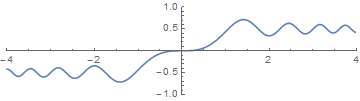In fact there is a formula for this solution: $x(t)=\int_0^t\sin\left(\frac{\pi t^2}{2}\right)\,dt$ This integral defines a specific function of $t$, since $\sin\left(\frac{\pi t^2}{2}\right)$ is a continuous function. However, the integral of this function cannot be expressed in terms of functions familiar to you from calculus class. (You can't "do" the integral.) Nevertheless, it is a genuine function and nowadays it has a name: $\text{FresnelS}(t):=\int_0^t\sin\left(\frac{\pi t^2}{2}\right)\,dt$ Giving it a name doesn't tell you how to calculate this function. Fresnel probably calculated values of the function using its power series.

## Symmetries

#### A symmetry is a function.

A symmetry is (roughly speaking) a way of changing an object so that it looks exactly the same after it is changed as before.

### Symmetries of a square

Below are three symmetries of a square. In each case the square is moved as shown. The labels show what the movement did to the corners. The symmetry moves the sides so that each side connects the same two corners. The labels are not part of the square.

#### Diagonal flip: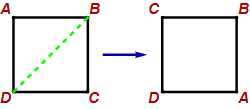#### Horizontal flip: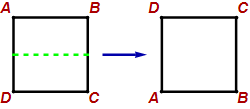#### Clockwise quarter turn: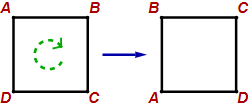There are eight symmetries of a square altogether, including the identity symmetry that leaves the square alone. These symmetries constitute the Dihedral group of order $8$.

### Representations of these permutations

Each symmetry can be represented in several different ways as permutations (bijective maps) of its corners. Representations of permutations are also presented in Representations of discrete functions.

#### Tables

These three symmetries may be represented as tables:

Diagonal flip: $\scriptstyle\begin{pmatrix} A&B&C&D\\ C&B&A&D \end{pmatrix}$

Horizontal flip: $\scriptstyle\begin{pmatrix} A&B&C&D\\ D&C&B&A \end{pmatrix}$

Clockwise quarter turn: $\scriptstyle\begin{pmatrix} A&B&C&D\\ B&C&D&A \end{pmatrix}$

#### Diagonal flip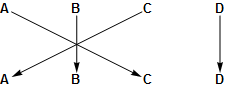#### Horizontal flip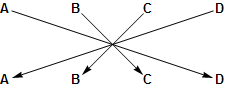#### Clockwise quarter turn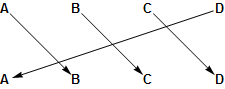#### Diagonal flip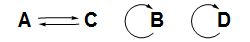#### Horizontal flip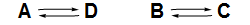#### Clockwise quarter turn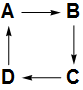All permutations of finite sets behave the way these do: The endograph is a union of disjoint cycles.

### Cycle notation

The disjoint cycle notation for permutations is particularly well adapted to composing permutations by hand.

Diagonal flip: $(A C)(B)(D)$

Horizontal flip: $(A D)(B C)$

Clockwise quarter turn: $(A B C D)$

This notation is intended be read this way: If $x$ has a parenthesis to its right, then $p(x)$ is the first symbol in the same parenthesized group, otherwise $p(x)$ is the next symbol to the right in the group. So given the permutation $p=(132)(8)(5467)$, $p(1)=3$, $p(2)=1$ and $p(8)=8$.

## Map

The idea that a function is a map
is one of the most powerful metaphors in mathematics.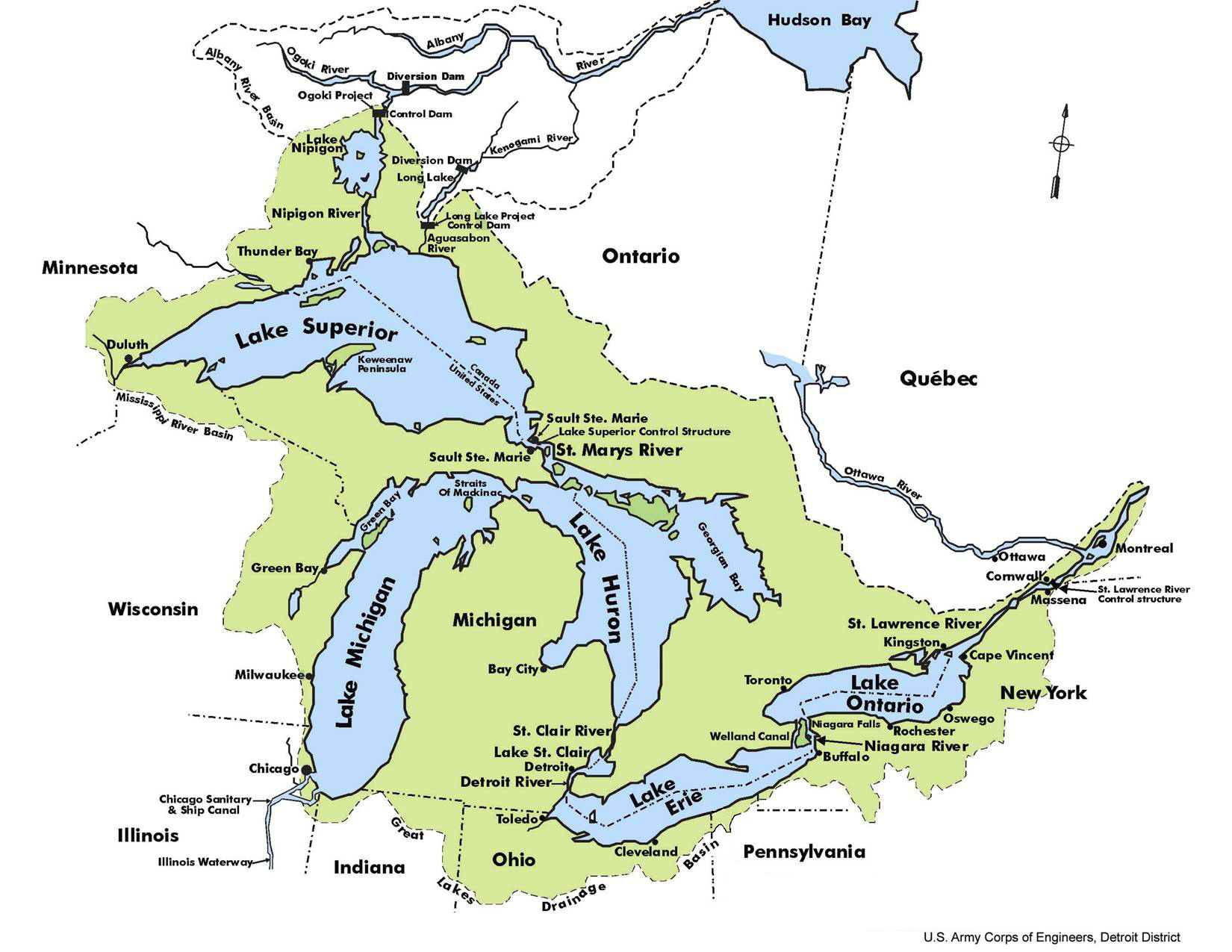This map is not a function, it is a physical object. But it is modeled as a mathematical function in this way:

• The domain of the map is an area on a sphere which is intended to model part of the surface of the Earth.
• The codomain is an area in the plane $\mathbb{R}\times\mathbb{R}$. The codomain is represented (approximately!) by the pixels on your screen.
• The map is a homeomorphism, which implies that it is bijective and continuous.
• Certain points and areas on the physical map are labeled in one way or another to give information about the area on the surface of the Earth that it refers to. In this particular map:
• The lakes are in blue and are named.
• The states and provinces are named and their boundaries are shown.
• The green area refers to the drainage basin of the Great Lakes.

The mathematical function corresponding to the map takes a point on the boundary of Michigan (for example) in the real world to a point on your screen. A blue point on the screen is a value of the function at a point in some actual real-world lake. All this is an idealization of the real map, which is necessarily only approximate.

Mapping the Earth's surface comes with lots of math dating back hundreds of years. Much of the work comes from the fact that a mathematical map from a sphere to a plane cannot simultaneously preserve both area and angles.

A decent physical map is more than continuous (taking nearby points on the Earth to nearby points on the screen):

• It is expected to approximately preserve the shapes of areas on the Earth (states, lakes, and so on).
• It is expected to allow you to calculate approximate distances on the Earth by measuring distances on the map.

### The fundamental property of the concept of "map"

What mathematicians call "maps" are not necessarily geographical maps. In math, we use the word "map" by analogy with the kind of map you see in an atlas:

If a mathematician calls a particular function a map,
then it preserves some property or structure or shape
that the mathematician is interested in.

The examples below illustrate some functions from the plane to itself that preserve area or angles but not both. In fact, a function from the plane to itself can preserve area and angles simultaneously (any rotation with determinant $1$ and any translation will do that), but a map from a sphere to a plane cannot do both at once.

#### Example: Functions preserving area

Any matrix with determinant $1$ preserves area. In each example, the function is $(x,y)\mapsto M.{x\choose y}$, where $M$ is the matrix shown. The diagram shows what the function does to three specific shapes.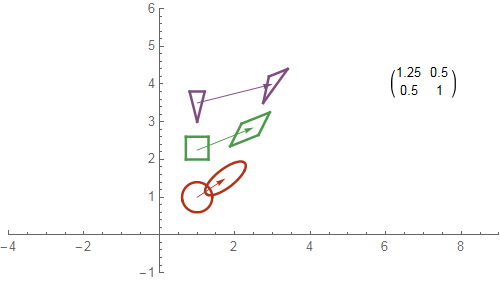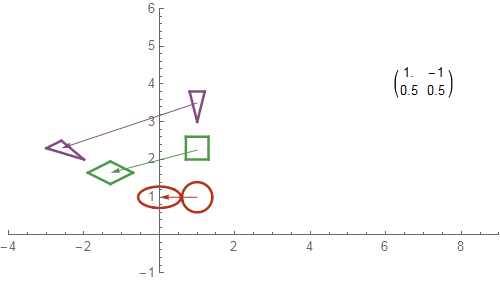#### Examples: Functions preserving angles

Any rotation matrix preserves angles. Such a function is called conformal.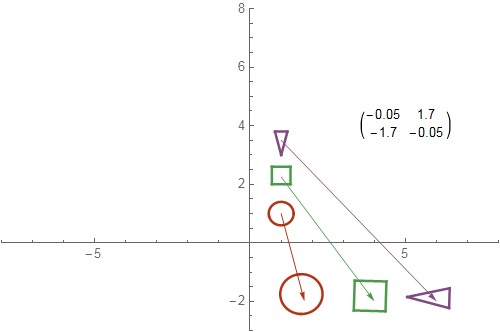There are more of these pictures in the Mathematica site on abstractmath.org. The pictures of angle-preserving functions are called epf#.gif or EqAng#.gif, where # is a digit, and the cographs are at epf#eg.gif or apf#ed.gif.

The Mathematica Demonstrations Project has many examples of linear transformations.

## Structure-preserving functions

#### A structure-preserving function preserves some specific structure on a math object.

A structure-preserving function is often called a map, because it "preserves" something of interest the way a physical map does. Two examples of this are given in the section on maps.

The notion of "preserves" is typically defined separately for different types of structure. A function that preserves an algebraic operation (as in the example immediately below) is called a homomorphism. Other words are used for other structures. It is also common to call any particular structure-preserving function a morphism.

#### Example

Let's define a function $h:\mathbb{R}\to\mathbb{R}$ by $f(x)=2x$. Now do some basic algebra: For any real numbers $r$ and $s$, $h(r+s)=2(r+s)=2r+2s=h(r)+h(s)$ For example, $h(3+5)=h(8)=16$ and $h(3)+h(5)=6+10=16$ We say $h$ preserves the structure of addition on the real numbers. This is typically expressed by the formula $h(r+s)=h(r)+h(s))$

It may not be clear why we call addition on the reals a structure. This is because algebra notation hides the structure in a very cramped notation designed to make it easy to do algebra with pencil and paper. We can express addition in a much more open way using trees. (There is more about this in my post Presenting binops as trees.)

The expression "$3+5$" is a symbol that can be exhibited this way: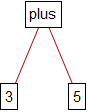In algebra we evaluate "$3+5$" by writing "$3+5=8$". Let us show this in a much more explicit way: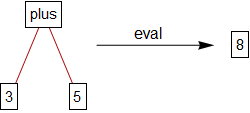Now I can express the fact that $h$ "preserves addition" using this diagram: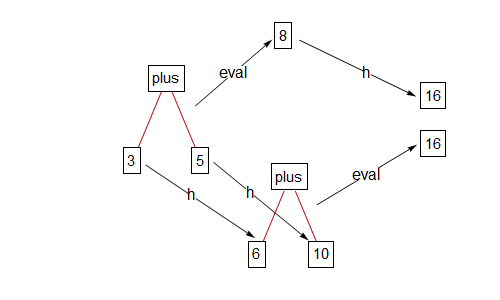If you start at the left side of the diagram and go to the right over the top, you apply $\text{eval}$ to get $8$, and then you apply $h$ to get $16$. If you go to the right using the lower route, you apply $h$ to each of $3$ and $5$ to get $6$ and $10$, and then you apply $\text{eval}$ to get $16$. Because you get the same thing going over or going under (for any real numbers, not just $3$ and $5$), we say the diagram commutes.

This meaning of the word "commutative" in "commutative diagram" is different from the meaning of the word used in a sentence such as "addition is commutative", meaning $a+b=b+a$. The connection between the two meanings is that in both cases, you can do something in two different ways and get the same answer.

#### Other examples

• For all real $x$ and $y$, $2^{x+y}=2^x2^y$. So the function $\text{exp}(x):=2^x$ takes addition on $\mathbb{R}$ to multiplication on $\mathbb{R}$, so that $\text{exp}$ is a homomorphism from addition to multiplication.
• The function $k(x):=x+3$ has the property that if $x\lt y$, then $k(x)\lt k(y)$; we say that $k$ is an order-preserving function.
• Differentiation, in other words $f\mapsto f'$, is an operator that takes a function to its derivative. Differentiation preserves addition; you learn in first semester calculus that $(f+g)'=f'+g'$.
• Note that because of the product rule, differentiation does not preserve multiplication of functions, and because of the chain rule, differentiation does not preserve composition of functions.
• This diagram (where $h(x)=2x$ as above) does not commute:
•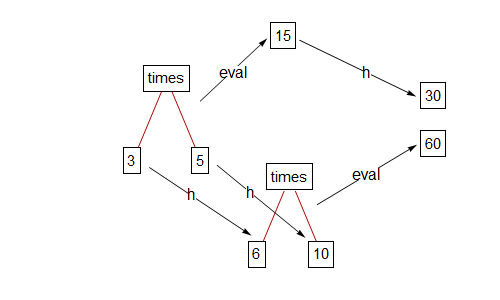So $h$ does not preserve multiplication.

• You can check that $s(x):=x^2$ does preserve multiplication (but not addition!).

Structure-preserving maps (morphisms)
occur in every branch of math.

### Category theory

Category theory is an axiomatization of the behavior of structure-preserving maps. It has become quite useful in many branches of math and it has revolutionized some of those branches.

Like all useful axiomatically-defined math structures, categories include objects that are quite different from the phenomena that motivated the definition. In particular, objects in a category don't have to be sets, much less structured sets, and morphisms don't have to be functions at all.

Sources available on line: Logic Matters: Category Theory.